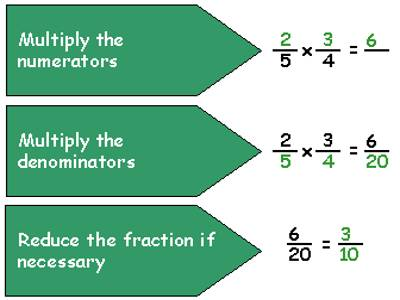Multiplying Fractions

To multiply fractions, first, we simplify the fractions if they are not in lowest terms. Then we multiply the numerators of the fractions to get the new numerator and multiply the denominators of the fractions to get the new denominator. Simplify the resulting fraction if possible.

To Multiply Fractions:

• Multiply the numerators of the fractions
• Multiply the denominators of the fractions
• Place the product of the numerators over the product of the denominators
• Simplify the FractionExample: Multiply 2/9 and 3/12.

• Multiply the numerators (2*3=6)
• Multiply the denominators (9*12=108)
• Place the product of the numerators over the product of the denominators (6/108)
• Simplify the Fraction (6/108 = 1/18)

The Easy Way, it is often simplest to “cancel” before doing the multiplication. Canceling is dividing one factor of the numerator and one factor of the denominator by the same number.

For example: 2/9 * 3/12 = (2*3)/(9*12) = (1*3)/(9*6) = (1*1)/(3*6) = 1/18.

Note that multiplying fractions is frequently expressed using the word “of.” For example, to find one-fifth of 10 pieces of candy, you would multiply 1/5 times 10, which equals 2. Study the example problems to see how to apply the rules for multiplying fractions.

Information Source;